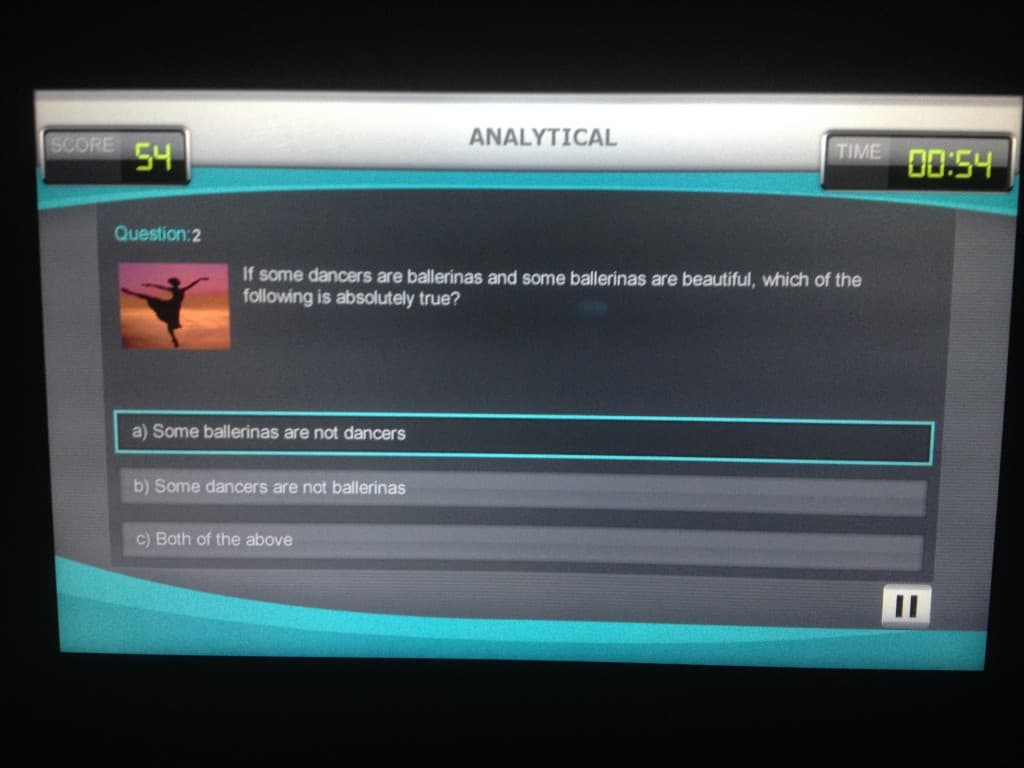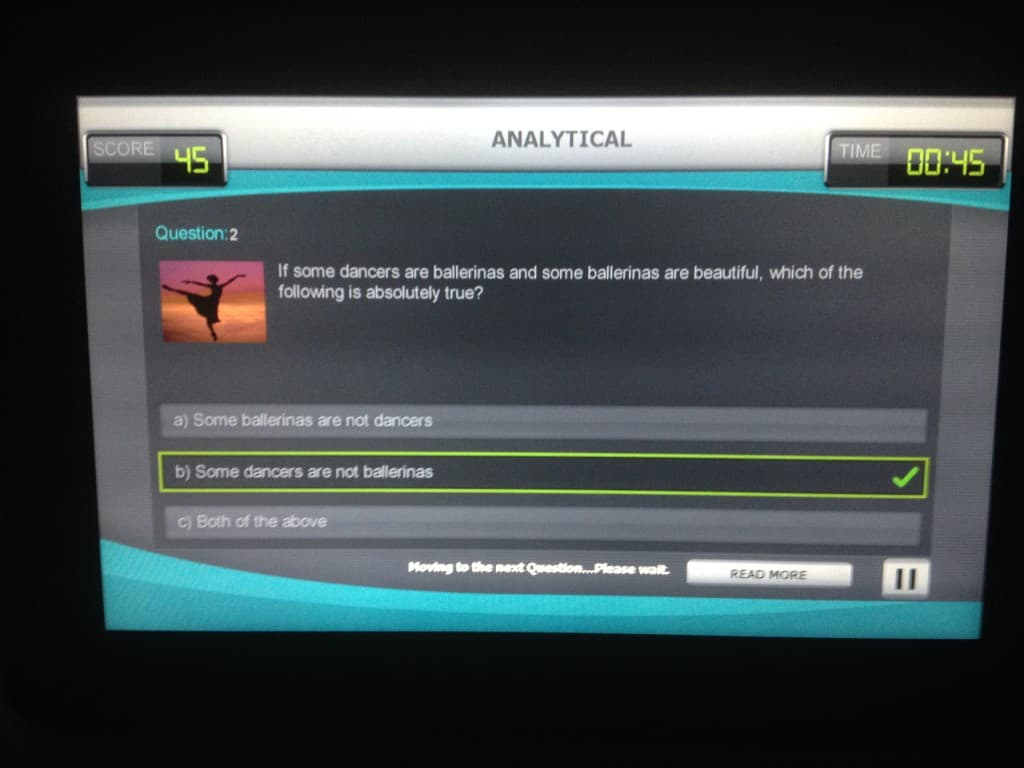# Too stupid for airplane games – syllogism versus logic

During the long flight from Narita to Vienna I spent some time with a game called “Brain Teaser”. Math, general knowledge was ok, but in the section of analytic I failed badly. Let us look at one of the questions:Now if you cannot read it, the question was:

If some dancers are ballerinas, and some ballerinas are beautiful, then which of the following statements is absolutely true:

a) Some ballerinas are not dancers.
b) Some dancers are not ballerinas.
c) Both of the above.

Now, in my understanding this is:

Assume
$\exists x (\mbox{dancer}(x) \rightarrow \mbox{ballerina}(x))$

(dropping the part on beautiful as it is irrelevant). And the answer part:

a) $$\exists x(\mbox{ballerina}(x) \rightarrow \lnot \mbox{dancer}(x))$$

b) $$\exists x(\mbox{dancer}(x) \rightarrow \lnot \mbox{ballerina}(x))$$

Now, taking the model of one dancer which is also ballerina (and to make everyone happy, also beautiful), then neither a) nor b) holds, but the assumption does.

Here is now the answer the game gave me:I guess logic and analytic does not go well together 🙁

Of course the answer is easy, and comes from the old time of syllogism, where categorical propositions are used. In this case some means a non-empty, proper subset of the whole. In this case, the answer is easy to understand.

So we see, studying logics is sometimes not so helpful!